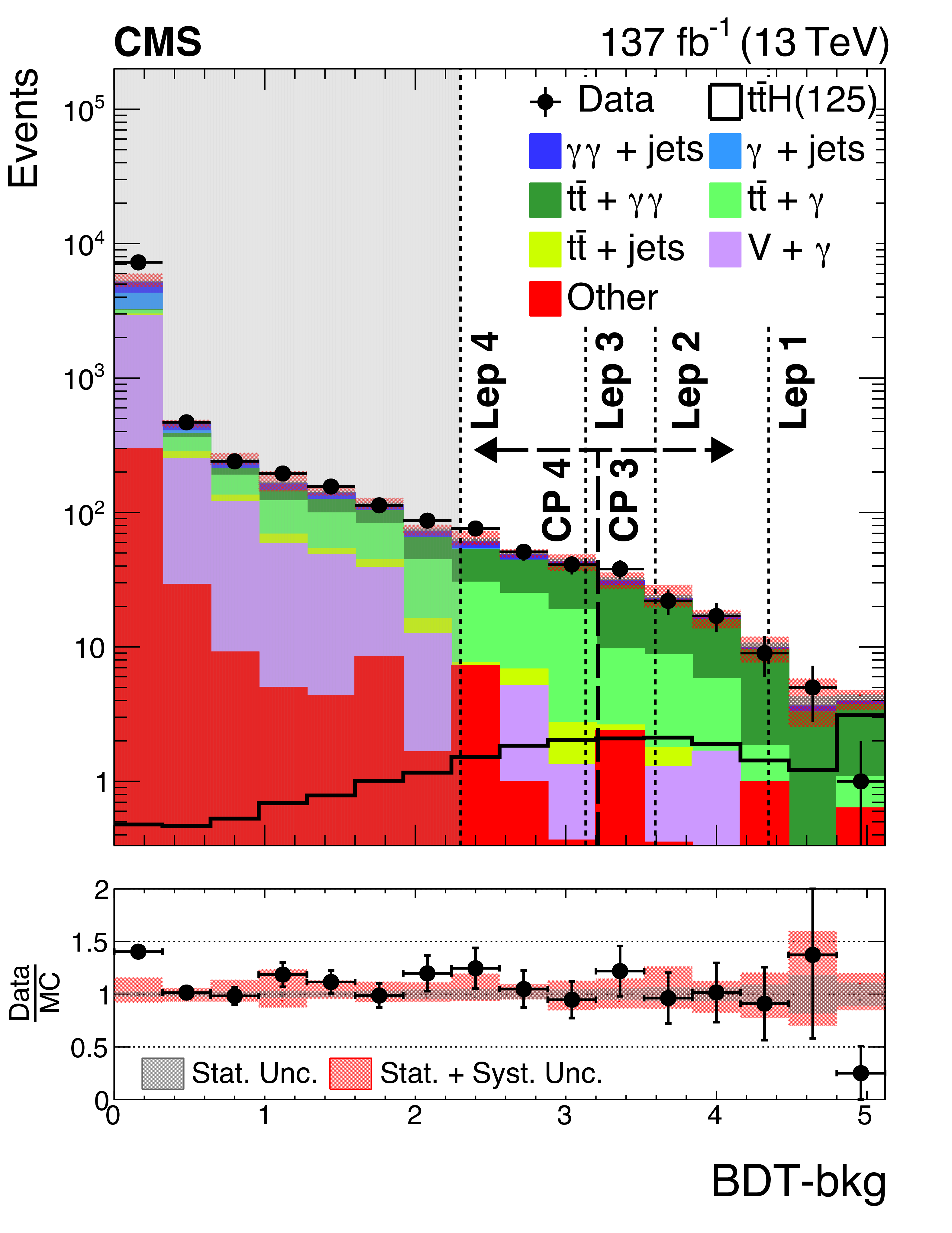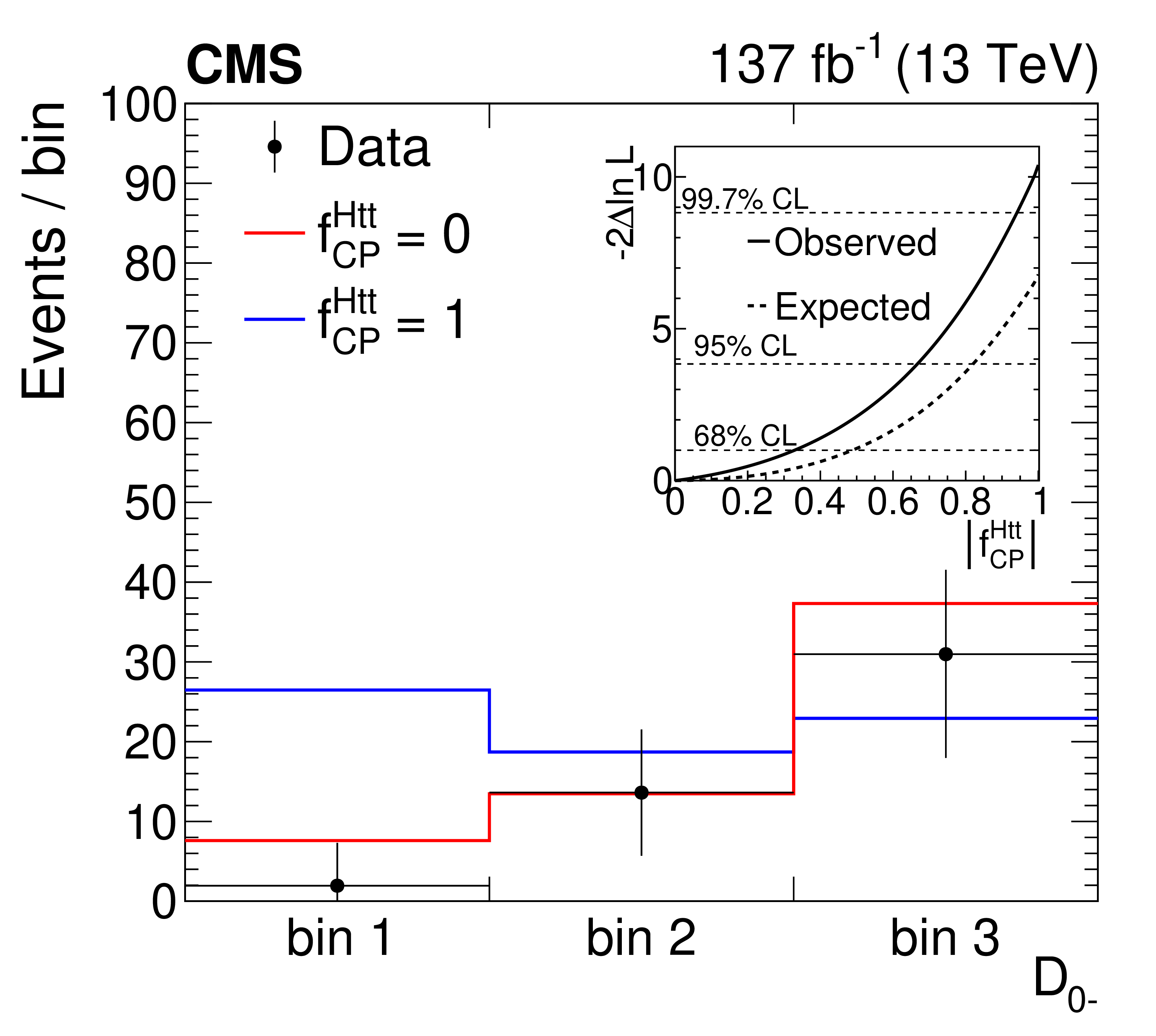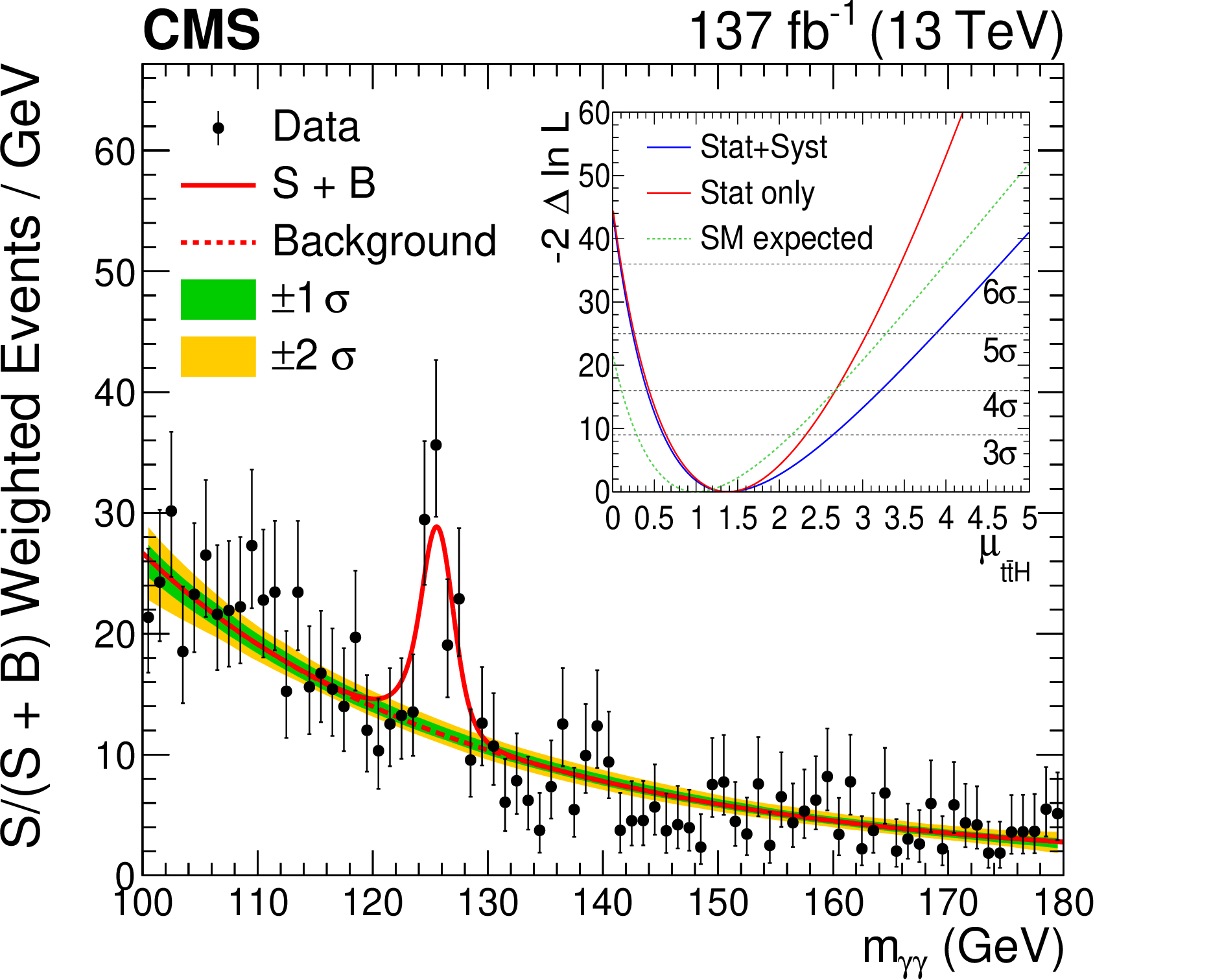•The CMS collaboration has achieved the first observation of a ttH process in a single Higgs boson decay channel. The Higgs boson is examined in its decay to two photons when produced alongside a top quark and antiquark. This enables the measurement of the interaction strength as well as a test of the charge-parity structure between the Higgs boson and top quark, an important property connected to the matter-antimatter asymmetry in the Universe.

Since the first observation of the Higgs boson by the ATLAS and CMS collaborations in 2012, understanding its properties has remained one of the highest priorities of the Large Hadron Collider (LHC) physics program. The Higgs boson plays a central role in our leading theory for describing the elementary particles and the interactions between them: the Standard Model of particle physics. The Standard Model was developed throughout the 20th century and reached its current form in the 1970s. It predicts the existence of the Higgs boson as a consequence of the electroweak symmetry breaking mechanism, through which elementary particles attain their masses.

The Standard Model is known to be an incomplete description of our universe. Even so, the discovery of the Higgs is among the greatest experimental achievements of the predictive power of the Standard Model but many of its properties have not been studied in detail. For example, does the Higgs boson interact with other elementary particles in the manner predicted by the Standard Model? Changes to those interactions can also be predicted by new, alternative, models that expand the Standard Model. That way, studying the properties of the Higgs boson provides an intriguing gateway for hints of physics beyond the Standard Model.

Some new physics models predict alternative mechanisms to generate mass that could significantly modify properties of the interactions between the Higgs boson and other elementary particles. For example, the strength of the interaction, which is called the “coupling strength”, would be different under new physics. The coupling strength between the Higgs boson and a given particle is predicted to be proportional to that particle's mass: the more massive the particle that the Higgs interacts with, the greater the coupling strength between them.

So far, all measurements of the properties of the Higgs boson have been in agreement with predictions made by the Standard Model. With the LHC collecting more data and the development of new analysis techniques, CMS physicists have been able to make more precise measurements of the Higgs boson's properties, including the “top quark Yukawa coupling''. This is the term given to the interaction strength between the Higgs boson and the top quark, where the latter is the most massive elementary particle of the Standard Model. A primary means of studying the top quark Yukawa coupling is through a process in which the Higgs boson is produced alongside a top quark and antiquark, called ttH production.

In addition to the coupling strength, the charge conjugation parity (CP) structure of an interaction is also of great interest to particle physicists. This collectively describes two types of symmetry: charge conjugation symmetry and parity symmetry. Charge conjugation symmetry implies that the laws of physics remain unchanged if a particle is exchanged with its antiparticle. Meanwhile, parity symmetry suggests that the laws of physics remain unchanged if a particle’s spatial coordinates (x, y, and z) are simultaneously inverted (to -x, -y and -z, respectively). When charge conjugation and parity symmetry hold, the CP structure is said to be “CP-even”. However, if they do not, CP violation is said to have occurred. The most extreme case is when the symmetry does not hold at all, in that case, the CP structure is said to be “CP-odd”.

The phenomenon of CP violation has been known to occur in the Standard Model, which provides a motivation for its study. CP violation could explain the matter-antimatter asymmetry of the universe. Our universe is dominated by matter, not antimatter -- our bodies are primarily composed of protons, neutrons, and electrons, rather than their antiparticles (antiprotons, antineutrons and positrons, respectively). Physicists do not yet know why there is this huge domination of matter over antimatter, nor do we know which mechanism enables this. The amount of CP violation currently observed in the Standard Model is insufficient to explain the matter-antimatter imbalance that is observed. Therefore, searching for additional sources of CP violation that are predicted in some new physics models may aid our understanding of this important unanswered question.

The CP structure of the top quark Yukawa coupling can be investigated through the behaviour of the final state decay products from ttH production. However, a challenge in identifying most LHC events is that most interesting particles decay almost instantaneously before they reach the CMS detector. This is also the case for the top quark and the Higgs boson, so ttH events must be identified through their decay products. In almost all cases, a top quark decays into a b-jet and a W boson. The latter can go on to decay into a pair of quarks or to a lepton and its neutrino. With two top quarks decaying each to a W boson and b-jet, top quark-antiquark pairs can create different signatures, with six jets (all hadronic), four jets and a charged lepton (semileptonic), or two charged leptons and two jets (dileptonic).  Similarly, there are many different decay channels for the Higgs, but this study considers a relatively rare decay of the Higgs boson: into two photons (a diphoton pair). Despite its rarity, studying this decay channel provides very precise measurements. The CMS electromagnetic calorimeter measures the energies of photons very precisely so the mass of a diphoton pair from the Higgs boson can be determined to great precision.

However, these decay products are not unique to the ttH process: other Standard Model processes can produce the same decay products. Known as “background” processes, these can trick us into thinking we have observed the process we are looking for (our “signal” process) when we have actually observed a background process instead. The diphoton final state of the Higgs has a relatively small number of background processes and background is further suppressed by requiring that the Higgs boson is produced simultaneously with top quarks.Figure 1: Distribution of BDT-background (BDT-bkg) output used for categorising events where the top quark decays via leptonic decay, with invariant masses either between 100 and 120 GeV or 130 and 180 GeV. Depending on the amount of expected background, the events are divided into categories that are represented by thinly dashed vertical lines. The boundaries for the CP measurement categories are represented by the thicker dashed lines. Events in the grey shaded region are not used in the analysis.

To discriminate between signal and background processes, a type of machine learning algorithm called a Boosted Decision Tree (BDT) was trained to distinguish between simulations of both the ttH process and the background processes. For a given event, where an event refers to when a process has happened in the detector, the algorithm provides a score that indicates how ttH-like the event is. Selecting events only with very high scores means that only very ttH-like events are considered, allowing for more precise measurements of the coupling strength and the CP structure. Figure 1 shows the output distributions of this machine learning algorithm for one of the decay modes of the top quark-antiquark pair. An additional BDT then was trained to measure if the pure ttH sample was more consistent with the CP-even or CP-odd symmetry of the top quark Yukawa coupling. Figure 2 shows the output of this machine learning algorithm.Figure 2: The distribution for the D0- discriminant that is sensitive to the CP structure of the Higgs boson, comparing data (black points) to the CP odd (blue) and CP even (red) scenarios. The data contains only events that have a high BDT-bkg score and an invariant mass of the two photons between 115 and 135 GeV. The smaller plot in the top right-hand corner indicates that the fractional contribution of the CP-odd component is consistent with zero. Events have been weighted by the ratio between the number signal events and the total number of signal and background events in the study.

In addition to the use of machine learning algorithms, the decay products of the Higgs boson also help to distinguish between ttH events and background events. The invariant mass of the pair of photons must consistent with the mass of the Higgs boson so, by examining the invariant mass of events that satisfy the requirements on the machine learning algorithms' score, a “peak” of events around the mass of the Higgs boson is clearly visible. This is shown in Figure 3. The new results show that the measured coupling strength between the Higgs boson and the top quark is consistent within statistical and systematic uncertainties with the prediction of the Standard Model. More precisely, the ratio of the measured production rate to the rate predicted by the Standard Model was calculated to be 1.38, with an uncertainty of about 40%. Similarly, the measured CP structure of ttH production was found to be CP-even, which is consistent with the Standard Model prediction, and the study is sensitive enough to claim evidence that a CP-odd top quark Yukawa coupling is excluded.Figure 3: Diphoton invariant mass distribution for the selected events (black points) weighted by the ratio of the signal events and the total number of events. A fitted solid red curve is shown for signal and background events, while the fitted dashed red curve represents only background events. The yellow and green band represents uncertainties in the fitted background, at one and two standard deviations respectively. The distribution in the top right is the likelihood scan for the ratio between the measured production rate and that predicted by the Standard Model. The likelihood scan shows that the result has a significance of 6.6 standard deviations, which is substantially higher than that required to claim a discovery (5 standard deviations).  Events have been weighted by the ratio between the number signal events and the total number of signal and background events in the study.

This unique study has helped to further develop our understanding of the Higgs boson’s properties and has, once again, reinforced the robustness of the Standard Model. The uncertainties on the measurement are still of the order of 40% and are dominated by the number of ttH events so adding new data will improve this measurement. The next LHC run planned to start in 2021, and the High-Luminosity LHC run starting in the mid-2020s, are expected to provide substantially more data and will allow even more precise measurement of the properties of the Higgs boson.